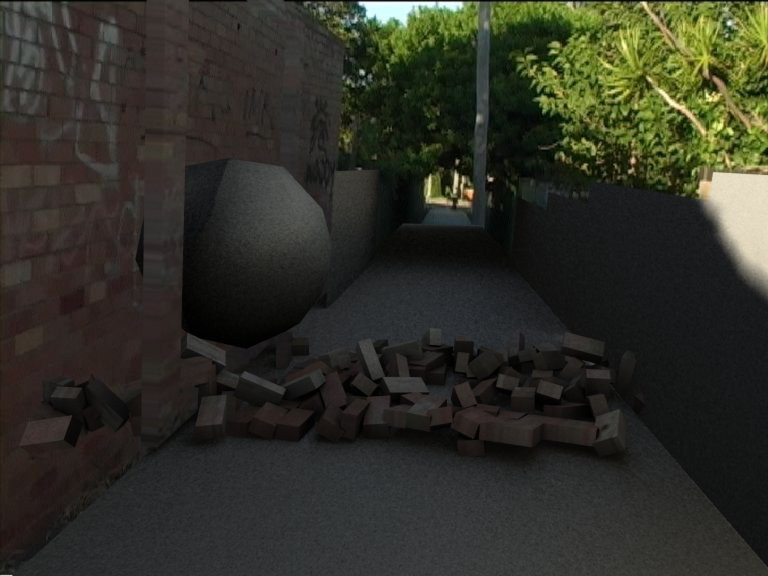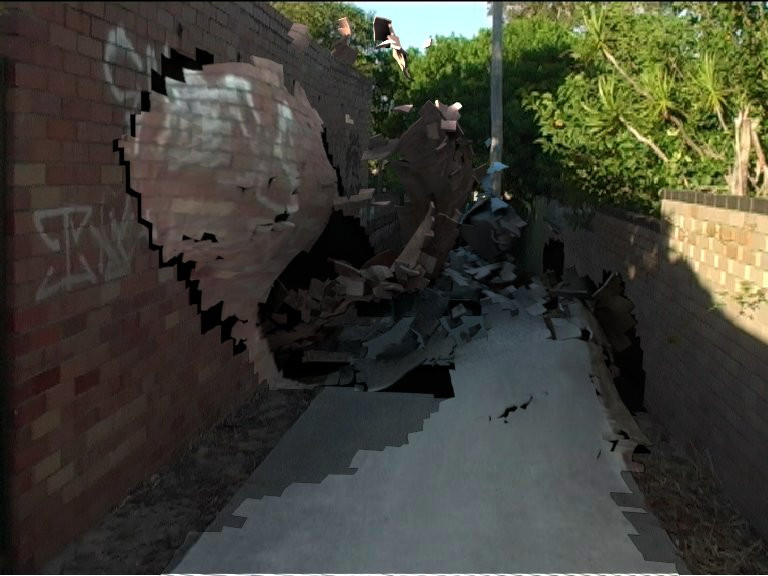# Dynamic camera mapping

The following is a brief writeup of what started as a simple camera mapping test in Houdini and later turned into a more involved implementation. I ended up creating a system that dynamically maps a texture on to an object depending on when it started moving, where it was in the frame and taking into account changing topology. Applications for this system include examples such as a static shot of a train approaching a camera, and then turning into ribbons and flying away- all of it done completely dynamically and procedurally. Basically anything that is in the frame can be mapped and deformed.

For the short of attention span, here is a render:

And here is what it looks like by itself:

The lighting is by no means great but that's not really the point.

OK so here is an early render where I camera mapped some brick things and smashed them out of the wall. Obviously you wouldn't want to apply a constant lighting model all the time, nor a lighting model itself- you need to blend from a constant shader to a lighting model based on how far the object is moving away from the wall. Turns out VOPs is great for this! length(Position-InitialPosition) = blending value.This is all fine and good for constant topology, but I want to dynamically rip the surface of the path up with the cloth solver. Why? Why not. I'm an intern, I get to explore these things! Plus the camera is moving over a wide range of motion, a static map from all the way at the end of the walk would look awful at the start- my reasoning therefore was that it would be better to dynamically apply the frames of the video as maps, WHEN a bit of geometry starts moving. This would give the optimum "matched" texture.

Here is my first test:I added a SOP solver to DOPs, and ran a test to check if a point had moved away from the ground plane (in VOPs, but later made a VEX node since if statements are pretty weird in VOPs), if it had then the UVs stopped updating for that point. It also stores the frame number at which this happened, this allows the shader to look up the correct texture to map to that poly and to calculate a blending value for the constant to lighting model change. I think later I made a distance attribute for a more accurate blend.

Here are the UVs being stuck down:If you look at the render with the texture applied, there is a problem in that you can't assign a texture on a per point or vertex basis, but a per poly basis. I'm actually taking all vertices on a poly and averaging the stored frame number attribute to get the right frame of video for that poly. A better solution would be to load all 4 possible textures for each quad, and blend (weighted by each vertex) across the poly.

Here's the initial test of that:So the theory checks out, and it's beginning to reduce the stepping you get from having a different texture applied to each poly. There's still a bit of that, but it's not as bad. Here's a pic of the interface and editing the shader- you can see the 4 possible textures to be loaded, and the custom inline VOP which compiles the string name from the 4d poly attribute holding the stored frame numbers.(I was using the poly on the left to view the order of vertex to texture assignment, if the order was incorrect each texture blend could be flipped on 4 possible axis)

The basic theory checks out, so I went ahead and refined the movement tester, writing this up in vex:

sop MovementTester(export vector uv = {0,0,0}; export float storeFnum = 0; int input_pid = 0) { int success = 0; float originalPtNumber = 0; vector originalPosition = {0,0,0}; //get original position of corresponding rest geometry point import("P", originalPosition, 1, input_pid); //calc the distance float theDistance = distance(P, originalPosition); //if the point has moved, store the frame number if(theDistance > 0.01 && storeFnum == 0) { storeFnum = Frame; } //if the point has NOT moved, grab the UV's from where it is "resting" if(theDistance < 0.01) { import("uv", uv, 1, input_pid); } }

this line: import("P", originalPosition, 1, input_pid); allows the node to pull in the original undeformed cloth geo on the second input of the VEX node, and then grab the point position of the relevant point (input_pid is a point attribute that the cloth system creates, it keeps track of the original pt number of a point on cloth- which will have changed due to changing topology via tearing).

Next up is to get a nice cloth sim, here I'm painting bits that I will allow to fly off:And it actually works! A combination of smaller polys, fixing a glitch that was resulting in a frame offset of 1 on the shader, and a few other things that I can't remember now, we get this: